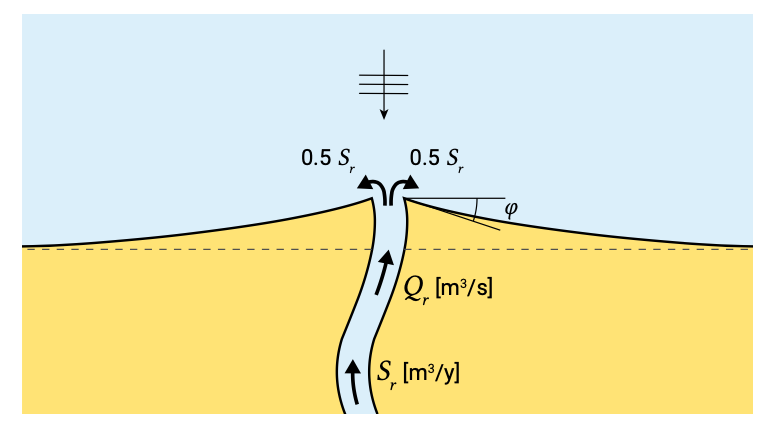# 8.4.6: Deltaic coastlines

$$\newcommand{\vecs}{\overset { \rightharpoonup} {\mathbf{#1}} }$$ $$\newcommand{\vecd}{\overset{-\!-\!\rightharpoonup}{\vphantom{a}\smash {#1}}}$$$$\newcommand{\id}{\mathrm{id}}$$ $$\newcommand{\Span}{\mathrm{span}}$$ $$\newcommand{\kernel}{\mathrm{null}\,}$$ $$\newcommand{\range}{\mathrm{range}\,}$$ $$\newcommand{\RealPart}{\mathrm{Re}}$$ $$\newcommand{\ImaginaryPart}{\mathrm{Im}}$$ $$\newcommand{\Argument}{\mathrm{Arg}}$$ $$\newcommand{\norm}{\| #1 \|}$$ $$\newcommand{\inner}{\langle #1, #2 \rangle}$$ $$\newcommand{\Span}{\mathrm{span}}$$ $$\newcommand{\id}{\mathrm{id}}$$ $$\newcommand{\Span}{\mathrm{span}}$$ $$\newcommand{\kernel}{\mathrm{null}\,}$$ $$\newcommand{\range}{\mathrm{range}\,}$$ $$\newcommand{\RealPart}{\mathrm{Re}}$$ $$\newcommand{\ImaginaryPart}{\mathrm{Im}}$$ $$\newcommand{\Argument}{\mathrm{Arg}}$$ $$\newcommand{\norm}{\| #1 \|}$$ $$\newcommand{\inner}{\langle #1, #2 \rangle}$$ $$\newcommand{\Span}{\mathrm{span}}$$$$\newcommand{\AA}{\unicode[.8,0]{x212B}}$$

Built primarily from river-borne sediments, deltas form when the amount of sediment delivered at the mouth of a river exceeds the amount removed by waves and tidal currents (see Ch. 2). The main effect of waves and tides is to redistribute the sediment along the coast. Section 2.7.3 has discussed the classification of deltas based on the relative river, wave and tidal influence.Figure 8.30: Developments of deltaic coastline with normally incident wave (no tidal influence). The river discharge is $$Q_r$$ and the sediment discharge $$S_r$$. The angle $$\phi$$ is determined by the sediment discharge and wave conditions.

Figure 8.30 shows the schematised development of a wave-dominated deltaic coastline (cf. Fig. 1.7). An equilibrium coastline position requires that all of the sediment supplied by the river is transported to the coastlines on either side of the river mouth by the wave-driven longshore currents. From the wave characteristics and the river sediment supply the equilibrium coastline orientation (see Fig. 8.30) can be calculated; at the delta mouth the angle $$\varphi$$ with the ‘normally’ incident waves will be such that the longshore transport rate is equal to $$\tfrac{1}{2} S_r$$.Figure 8.31: Delta erosion after the sediment supply is cut-off. Due to wave-induced longshore transport gradients sediments are redistributed along the coast, resulting in erosion of the delta (cf. the development of a shoreline perturbation in Sect. 8.4.4).

Man-induced changes in the characteristics of the river (e.g. sand mining in the river bed, or damming of the river for irrigation or hydro-power purposes), might change the natural accreting tendency of the coast to an eroding tendency in the vicinity of the river outlet (see Fig. 8.31).